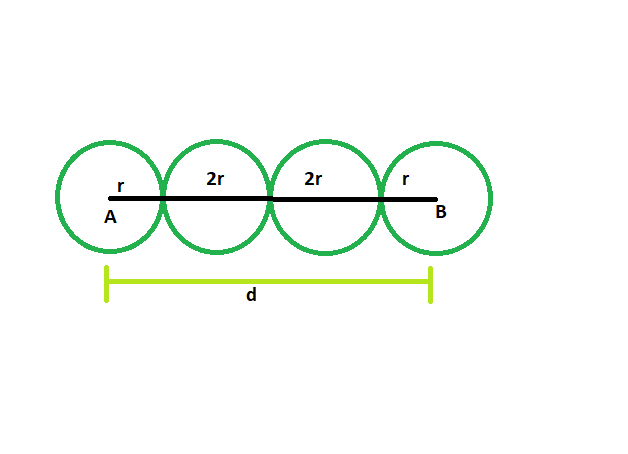# Find the radii of the circles which are lined in a row, and distance between the centers of first and last circle is given

Given here are n circles which touch each other externally, and are lined up in a row. The distance between the centers of the first and last circle is given. The circles have a radius of equal length. The task is to find the radius of each circle.

Examples:

```Input: d = 42, n = 4
Output: The radius of each circle is 7

Input: d = 64, n = 5
Output: The radius of each circle is 8
```Approach:
Suppose there are n circles each having radius of length r.
Let, the distance between the first and last circles = d
From the figure, it is clear,
r + r + (n-2)*2r = d
2r + 2nr – 4r = d
2nr – 2r = d

so, r = d/(2n-2)## C++

 `// C++ program to find radii of the circles ` `// which are lined in a row ` `// and distance between the ` `// centers of first and last circle is given ` ` `  `#include ` `using` `namespace` `std; ` ` `  `void` `radius(``int` `n, ``int` `d) ` `{ ` `    ``cout << ``"The radius of each circle is "` `         ``<< d / (2 * n - 2) << endl; ` `} ` ` `  `// Driver code ` `int` `main() ` `{ ` `    ``int` `d = 42, n = 4; ` `    ``radius(n, d); ` `    ``return` `0; ` `} `

## Java

 `// Java program to find radii of the circles ` `// which are lined in a row ` `// and distance between the ` `// centers of first and last circle is given ` `import` `java.io.*; ` ` `  `class` `GFG ` `{ ` ` `  `static` `void` `radius(``int` `n, ``int` `d) ` `{ ` `    ``System.out.print( ``"The radius of each circle is "` `        ``+d / (``2` `* n - ``2``)); ` `} ` ` `  `// Driver code ` `static` `public` `void` `main (String []args) ` `{ ` `    ``int` `d = ``42``, n = ``4``; ` `    ``radius(n, d); ` `} ` `} ` ` `  `// This code is contributed by anuj_67.. `

## Python3

 `# Python program to find radii of the circles ` `# which are lined in a row ` `# and distance between the ` `# centers of first and last circle is given ` ` `  `def` `radius(n, d): ` `    ``print``(``"The radius of each circle is "``, ` `        ``d ``/` `(``2` `*` `n ``-` `2``)); ` ` `  `d ``=` `42``; n ``=` `4``; ` `radius(n, d); ` ` `  ` `  `# This code is contributed by PrinciRaj1992 `

## C#

 `// C# program to find radii of the circles ` `// which are lined in a row ` `// and distance between the ` `// centers of first and last circle is given ` `using` `System; ` ` `  `class` `GFG ` `{ ` ` `  `static` `void` `radius(``int` `n, ``int` `d) ` `{ ` `    ``Console.Write( ``"The radius of each circle is "` `        ``+d / (2 * n - 2)); ` `} ` ` `  `// Driver code ` `static` `public` `void` `Main () ` `{ ` `    ``int` `d = 42, n = 4; ` `    ``radius(n, d); ` `} ` `} ` ` `  `// This code is contributed by anuj_67.. `

Output:

```The radius of each circle is 7
```

My Personal Notes arrow_drop_upCheck out this Author's contributed articles.

If you like GeeksforGeeks and would like to contribute, you can also write an article using contribute.geeksforgeeks.org or mail your article to contribute@geeksforgeeks.org. See your article appearing on the GeeksforGeeks main page and help other Geeks.

Please Improve this article if you find anything incorrect by clicking on the "Improve Article" button below.

Improved By : vt_m, princiraj1992

Article Tags :
Practice Tags :

Be the First to upvote.

Please write to us at contribute@geeksforgeeks.org to report any issue with the above content.